x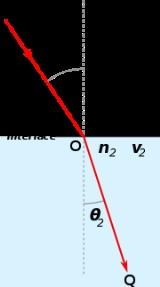Snell's lawEncyclopedia
In optics
Optics
Optics is the branch of physics which involves the behavior and properties of light, including its interactions with matter and the construction of instruments that use or detect it. Optics usually describes the behavior of visible, ultraviolet, and infrared light...

and physics
Physics
Physics is a natural science that involves the study of matter and its motion through spacetime, along with related concepts such as energy and force. More broadly, it is the general analysis of nature, conducted in order to understand how the universe behaves.Physics is one of the oldest academic...

, Snell's law (also known as Descartes' law, the Snell–Descartes law, and the law of refraction) is a formula used to describe the relationship between the angles of incidence
Angle of incidence
Angle of incidence is a measure of deviation of something from "straight on", for example:* in the approach of a ray to a surface, or* the angle at which the wing or horizontal tail of an airplane is installed on the fuselage, measured relative to the axis of the fuselage.-Optics:In geometric...

and refraction
Refraction
Refraction is the change in direction of a wave due to a change in its speed. It is essentially a surface phenomenon . The phenomenon is mainly in governance to the law of conservation of energy. The proper explanation would be that due to change of medium, the phase velocity of the wave is changed...

, when referring to light or other wave
Wave
In physics, a wave is a disturbance that travels through space and time, accompanied by the transfer of energy.Waves travel and the wave motion transfers energy from one point to another, often with no permanent displacement of the particles of the medium—that is, with little or no associated mass...

s passing through a boundary between two different isotropic media
Medium (optics)
An optical medium is material through which electromagnetic waves propagate. It is a form of transmission medium. The permittivity and permeability of the medium define how electromagnetic waves propagate in it...

, such as water and glass.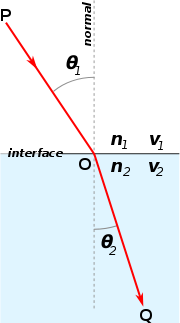In optics, the law is used in ray tracing
Ray tracing (physics)
In physics, ray tracing is a method for calculating the path of waves or particles through a system with regions of varying propagation velocity, absorption characteristics, and reflecting surfaces. Under these circumstances, wavefronts may bend, change direction, or reflect off surfaces,...

to compute the angles of incidence or refraction, and in experimental optics and gemology
Gemology
Gemology or gemmology is the science dealing with natural and artificial gems and gemstones. It is considered a geoscience and a branch of mineralogy...

to find the refractive index
Refractive index
In optics the refractive index or index of refraction of a substance or medium is a measure of the speed of light in that medium. It is expressed as a ratio of the speed of light in vacuum relative to that in the considered medium....

of a material. The law is also satisfied in metamaterials, which allow light to be bent "backward" at a negative angle of refraction (negative refractive index).

Although named after Dutch astronomer Willebrord Snellius
Willebrord Snellius
Willebrord Snellius was a Dutch astronomer and mathematician. In the west, especially the English speaking countries, his name has been attached to the law of refraction of light for several centuries, but it is now known that this law was first discovered by Ibn Sahl in 984...

(1580–1626), the law was first accurately described by the Persian scientist Ibn Sahl
Ibn Sahl
This article is about the physicist. For the physician, see Ali ibn Sahl Rabban al-Tabari. For the poet, see Ibn Sahl of Sevilla.Ibn Sahl was a Muslim Persian mathematician, physicist and optics engineer of the Islamic Golden Age associated with the Abbasid court of Baghdad...

at Baghdad
Baghdad
Baghdad is the capital of Iraq, as well as the coterminous Baghdad Governorate. The population of Baghdad in 2011 is approximately 7,216,040...

court, when in 984 he used the law to derive lens shapes that focus light with no geometric aberrations in the manuscript On Burning Mirrors and Lenses (984).

Snell's law states that the ratio of the sine
Sine
In mathematics, the sine function is a function of an angle. In a right triangle, sine gives the ratio of the length of the side opposite to an angle to the length of the hypotenuse.Sine is usually listed first amongst the trigonometric functions....

s of the angles of incidence and refraction is equivalent to the ratio of phase velocities
Phase velocity
The phase velocity of a wave is the rate at which the phase of the wave propagates in space. This is the speed at which the phase of any one frequency component of the wave travels. For such a component, any given phase of the wave will appear to travel at the phase velocity...

in the two media, or equivalent to the opposite ratio of the indices of refraction: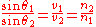with each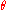as the angle measured from the normal,as the velocity of light in the respective medium (SI units are meters per second, or m/s) andas the refractive index (which is unitless) of the respective medium.

The law follows from Fermat's principle of least time
Fermat's principle
In optics, Fermat's principle or the principle of least time is the principle that the path taken between two points by a ray of light is the path that can be traversed in the least time. This principle is sometimes taken as the definition of a ray of light...

, which in turn follows from the propagation of light as waves.

## History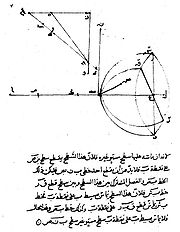Ptolemy
Ptolemy
Claudius Ptolemy , was a Roman citizen of Egypt who wrote in Greek. He was a mathematician, astronomer, geographer, astrologer, and poet of a single epigram in the Greek Anthology. He lived in Egypt under Roman rule, and is believed to have been born in the town of Ptolemais Hermiou in the...

, a Greek living in Alexandria
Alexandria
Alexandria is the second-largest city of Egypt, with a population of 4.1 million, extending about along the coast of the Mediterranean Sea in the north central part of the country; it is also the largest city lying directly on the Mediterranean coast. It is Egypt's largest seaport, serving...

, had found a relationship regarding refraction angles, but it was inaccurate for angles that were not small. Ptolemy was confident he had found an accurate empirical law, partially as a result of fudging his data to fit theory (see: confirmation bias
Confirmation bias
Confirmation bias is a tendency for people to favor information that confirms their preconceptions or hypotheses regardless of whether the information is true.David Perkins, a geneticist, coined the term "myside bias" referring to a preference for "my" side of an issue...

). Alhazen, in his Book of Optics
Book of Optics
The Book of Optics ; ; Latin: De Aspectibus or Opticae Thesaurus: Alhazeni Arabis; Italian: Deli Aspecti) is a seven-volume treatise on optics and other fields of study composed by the medieval Muslim scholar Alhazen .-See also:* Science in medieval Islam...

(1021), came closer to discovering the law of refraction, though he did not take this step.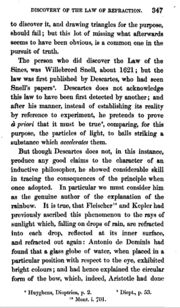The law of refraction was first accurately described by Ibn Sahl
Ibn Sahl
This article is about the physicist. For the physician, see Ali ibn Sahl Rabban al-Tabari. For the poet, see Ibn Sahl of Sevilla.Ibn Sahl was a Muslim Persian mathematician, physicist and optics engineer of the Islamic Golden Age associated with the Abbasid court of Baghdad...

, of Baghdad
Baghdad
Baghdad is the capital of Iraq, as well as the coterminous Baghdad Governorate. The population of Baghdad in 2011 is approximately 7,216,040...

, in the manuscript On Burning Mirrors and Lenses (984). He made use of it to work out the shapes of lenses that focus light with no geometric aberrations, known as anaclastic lenses.

The law was rediscovered by Thomas Harriot
Thomas Harriot
Thomas Harriot was an English astronomer, mathematician, ethnographer, and translator. Some sources give his surname as Harriott or Hariot or Heriot. He is sometimes credited with the introduction of the potato to Great Britain and Ireland...

in 1602, who however did not publish his results although he had corresponded with Kepler on this very subject. In 1621, Willebrord Snellius
Willebrord Snellius
Willebrord Snellius was a Dutch astronomer and mathematician. In the west, especially the English speaking countries, his name has been attached to the law of refraction of light for several centuries, but it is now known that this law was first discovered by Ibn Sahl in 984...

(Snel) derived a mathematically equivalent form, that remained unpublished during his lifetime. René Descartes
René Descartes
René Descartes ; was a French philosopher and writer who spent most of his adult life in the Dutch Republic. He has been dubbed the 'Father of Modern Philosophy', and much subsequent Western philosophy is a response to his writings, which are studied closely to this day...

independently derived the law using heuristic momentum conservation arguments in terms of sines in his 1637 treatise Discourse on Method
Discourse on Method
The Discourse on the Method is a philosophical and autobiographical treatise published by René Descartes in 1637. Its full name is Discourse on the Method of Rightly Conducting One's Reason and of Seeking Truth in the Sciences .The Discourse on Method is best known...

, and used it to solve a range of optical problems. Rejecting Descartes' solution, Pierre de Fermat arrived at the same solution based solely on his principle of least time.

According to Dijksterhuis, "In De natura lucis et proprietate (1662) Isaac Vossius said that Descartes had seen Snell's paper and concocted his own proof. We now know this charge to be undeserved but it has been adopted many times since." Both Fermat and Huygens repeated this accusation that Descartes had copied Snell.

In French
French language
French is a Romance language spoken as a first language in France, the Romandy region in Switzerland, Wallonia and Brussels in Belgium, Monaco, the regions of Quebec and Acadia in Canada, and by various communities elsewhere. Second-language speakers of French are distributed throughout many parts...

, Snell's Law is called "la loi de Descartes" or "loi de Snell-Descartes."In his 1678 Traité de la Lumiere, Christiaan Huygens showed how Snell's law of sines could be explained by, or derived from, the wave nature of light, using what we have come to call the Huygens–Fresnel principle.

## Explanation

Snell's law is used to determine the direction of light rays through refractive media with varying indices of refraction. The indices of refraction of the media, labeled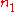,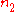and so on, are used to represent the factor by which a light ray's speed decreases when traveling through a refractive medium, such as glass or water, as opposed to its velocity in a vacuum.

As light passes the border between media, depending upon the relative refractive indices of the two media, the light will either be refracted to a lesser angle, or a greater one. These angles are measured with respect to the normal line, represented perpendicular to the boundary. In the case of light traveling from air into water, light would be refracted towards the normal line, because the light is slowed down in water; light traveling from water to air would refract away from the normal line.

Refraction between two surfaces is also referred to as reversible because if all conditions were identical, the angles would be the same for light propagating in the opposite direction.

Snell's law is generally true only for isotropic or specular media (such as glass
Glass
Glass is an amorphous solid material. Glasses are typically brittle and optically transparent.The most familiar type of glass, used for centuries in windows and drinking vessels, is soda-lime glass, composed of about 75% silica plus Na2O, CaO, and several minor additives...

). In anisotropic media such as some crystal
Crystal
A crystal or crystalline solid is a solid material whose constituent atoms, molecules, or ions are arranged in an orderly repeating pattern extending in all three spatial dimensions. The scientific study of crystals and crystal formation is known as crystallography...

s, birefringence
Birefringence
Birefringence, or double refraction, is the decomposition of a ray of light into two rays when it passes through certain anisotropic materials, such as crystals of calcite or boron nitride. The effect was first described by the Danish scientist Rasmus Bartholin in 1669, who saw it in calcite...

may split the refracted ray into two rays, the ordinary or o-ray which follows Snell's law, and the other extraordinary or e-ray which may not be co-planar with the incident ray.

When the light or other wave involved is monochromatic, that is, of a single frequency, Snell's law can also be expressed in terms of a ratio of wavelengths in the two media, λ1 and λ2: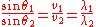## Derivations and formulae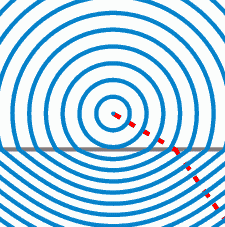Snell's law may be derived from Fermat's principle
Fermat's principle
In optics, Fermat's principle or the principle of least time is the principle that the path taken between two points by a ray of light is the path that can be traversed in the least time. This principle is sometimes taken as the definition of a ray of light...

, which states that the light travels the path which takes the least time. By taking the derivative
Derivative
In calculus, a branch of mathematics, the derivative is a measure of how a function changes as its input changes. Loosely speaking, a derivative can be thought of as how much one quantity is changing in response to changes in some other quantity; for example, the derivative of the position of a...

of the optical path length
Optical path length
In optics, optical path length or optical distance is the product of the geometric length of the path light follows through the system, and the index of refraction of the medium through which it propagates. A difference in optical path length between two paths is often called the optical path...

, the stationary point
Stationary point
In mathematics, particularly in calculus, a stationary point is an input to a function where the derivative is zero : where the function "stops" increasing or decreasing ....

is found giving the path taken by the light (though it should be noted that the result does not show light taking the least time path, but rather one that is stationary with respect to small variations as there are cases where light actually takes the greatest time path, as in a spherical mirror). In a classic analogy, the area of lower refractive index
Refractive index
In optics the refractive index or index of refraction of a substance or medium is a measure of the speed of light in that medium. It is expressed as a ratio of the speed of light in vacuum relative to that in the considered medium....

is replaced by a beach, the area of higher refractive index
Refractive index
In optics the refractive index or index of refraction of a substance or medium is a measure of the speed of light in that medium. It is expressed as a ratio of the speed of light in vacuum relative to that in the considered medium....

by the sea, and the fastest way for a rescuer on the beach to get to a drowning
Drowning
Drowning is death from asphyxia due to suffocation caused by water entering the lungs and preventing the absorption of oxygen leading to cerebral hypoxia....

person in the sea is to run along a path that follows Snell's law.

Alternatively, Snell's law can be derived using interference of all possible paths of light wave from source to observer—it results in destructive interference everywhere except extrema of phase (where interference is constructive)—which become actual paths.

Another way to derive Snell’s Law involves an application of the general boundary conditions of Maxwell equations for electromagnetic radiation
Electromagnetic radiation
Electromagnetic radiation is a form of energy that exhibits wave-like behavior as it travels through space...

.

Yet another way to derive Snell's law is based on translation symmetry considerations. For example, a homogeneous surface perpendicular to the z direction can not change the transverse momentum. Since the propagation vector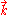is proportional to the photon's momentum, the transverse propagation direction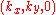must remain the same in both regions. Assuming without loss of generality a plane of incidence in the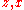plane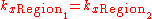. Using the well known dependence of the wave number on the refractive index
Refractive index
In optics the refractive index or index of refraction of a substance or medium is a measure of the speed of light in that medium. It is expressed as a ratio of the speed of light in vacuum relative to that in the considered medium....

of the medium, we derive Snell's law immediately.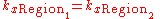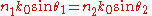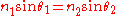where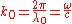is the wavenumber in vacuum. Note that no surface is truly homogeneous, in the least at the atomic scale. Yet full translational symmetry is an excellent approximation whenever the region is homogeneous on the scale of the light wavelength.

### Vector form

Given a normalized light vector l (pointing from the light source toward the surface) and a normalized plane normal vector n, one can work out the normalized reflected and refracted rays: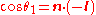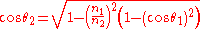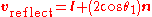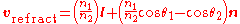Note: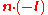must be positive. Otherwise, use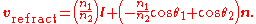Example: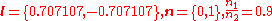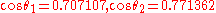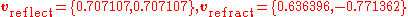The cosines may be recycled and used in the Fresnel equations
Fresnel equations
The Fresnel equations , deduced by Augustin-Jean Fresnel , describe the behaviour of light when moving between media of differing refractive indices...

for working out the intensity of the resulting rays.

Total internal reflection
Total internal reflection
Total internal reflection is an optical phenomenon that happens when a ray of light strikes a medium boundary at an angle larger than a particular critical angle with respect to the normal to the surface. If the refractive index is lower on the other side of the boundary and the incident angle is...

is indicated by a negative radicand in the equation for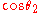. In this case, an evanescent wave
Evanescent wave
An evanescent wave is a nearfield standing wave with an intensity that exhibits exponential decay with distance from the boundary at which the wave was formed. Evanescent waves are a general property of wave-equations, and can in principle occur in any context to which a wave-equation applies...

is produced, which rapidly decays from the surface into the second medium. Conservation of energy is maintained by the circulation of energy across the boundary, averaging to zero net energy transmission.

## Total internal reflection and critical angle

When light travels from a medium with a higher refractive index to one with a lower refractive index, Snell's law seems to require in some cases (whenever the angle of incidence is large enough) that the sine of the angle of refraction be greater than one. This of course is impossible, and the light in such cases is completely reflected by the boundary, a phenomenon known as total internal reflection
Total internal reflection
Total internal reflection is an optical phenomenon that happens when a ray of light strikes a medium boundary at an angle larger than a particular critical angle with respect to the normal to the surface. If the refractive index is lower on the other side of the boundary and the incident angle is...

. The largest possible angle of incidence which still results in a refracted ray is called the critical angle; in this case the refracted ray travels along the boundary between the two media.

For example, consider a ray of light moving from water to air with an angle of incidence of 50°. The refractive indices of water and air are approximately 1.333 and 1, respectively, so Snell's law gives us the relation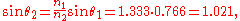which is impossible to satisfy. The critical angle θcrit is the value of θ1 for which θ2 equals 90°: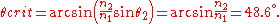## Dispersion

In many wave-propagation media, wave velocity changes with frequency or wavelength of the waves; this is true of light propagation in most transparent substances other than a vacuum. These media are called dispersive. The result is that the angles determined by Snell's law also depend on frequency or wavelength, so that a ray of mixed wavelengths, such as white light, will spread or disperse. Such dispersion of light in glass or water underlies the origin of rainbow
Rainbow
A rainbow is an optical and meteorological phenomenon that causes a spectrum of light to appear in the sky when the Sun shines on to droplets of moisture in the Earth's atmosphere. It takes the form of a multicoloured arc...

s and other optical phenomena, in which different wavelengths appear as different colors.

In optical instruments, dispersion leads to chromatic aberration
Chromatic aberration
In optics, chromatic aberration is a type of distortion in which there is a failure of a lens to focus all colors to the same convergence point. It occurs because lenses have a different refractive index for different wavelengths of light...

; a color-dependent blurring that sometimes is the resolution-limiting effect. This was especially true in refracting telescope
Refracting telescope
A refracting or refractor telescope is a type of optical telescope that uses a lens as its objective to form an image . The refracting telescope design was originally used in spy glasses and astronomical telescopes but is also used for long focus camera lenses...

s, before the invention of achromatic
Achromatic lens
An achromatic lens or achromat is a lens that is designed to limit the effects of chromatic and spherical aberration. Achromatic lenses are corrected to bring two wavelengths into focus in the same plane....

objective lenses.

## Lossy, absorbing, or conducting media

In a conducting medium, permittivity and index of refraction are complex-valued. Consequently, so are the angle of refraction and the wave-vector. This implies that, while the surfaces of constant real phase are planes whose normals make an angle equal to the angle of refraction with the interface normal, the surfaces of constant amplitude, in contrast, are planes parallel to the interface itself. Since these two planes do not in general coincide with each other, the wave is said to be inhomogeneous. The refracted wave is exponentially attenuated, with exponent proportional to the imaginary component of the index of refraction.

## See also

• Evanescent wave
Evanescent wave
An evanescent wave is a nearfield standing wave with an intensity that exhibits exponential decay with distance from the boundary at which the wave was formed. Evanescent waves are a general property of wave-equations, and can in principle occur in any context to which a wave-equation applies...

• Fresnel equations
Fresnel equations
The Fresnel equations , deduced by Augustin-Jean Fresnel , describe the behaviour of light when moving between media of differing refractive indices...

• Reflection (physics)
Reflection (physics)
Reflection is the change in direction of a wavefront at an interface between two differentmedia so that the wavefront returns into the medium from which it originated. Common examples include the reflection of light, sound and water waves...

• Refraction
Refraction
Refraction is the change in direction of a wave due to a change in its speed. It is essentially a surface phenomenon . The phenomenon is mainly in governance to the law of conservation of energy. The proper explanation would be that due to change of medium, the phase velocity of the wave is changed...

• Snell's window
Snell's window
Snell's window is a phenomenon by which an underwater viewer sees everything above the surface through a cone of light of width of about 96 degrees. This phenomenon is caused by refraction of light entering water, and is governed by Snell's Law...

• Total internal reflection
Total internal reflection
Total internal reflection is an optical phenomenon that happens when a ray of light strikes a medium boundary at an angle larger than a particular critical angle with respect to the normal to the surface. If the refractive index is lower on the other side of the boundary and the incident angle is...

• Calculus of variations
• Brachistochrone curve
Brachistochrone curve
A Brachistochrone curve , or curve of fastest descent, is the curve between two points that is covered in the least time by a point-like body that starts at the first point with zero speed and is constrained to move along the curve to the second point, under the action of constant gravity and...

for a simple proof by Jacob Bernoulli
• Hamiltonian optics
Hamiltonian optics
Lagrangian optics and Hamiltonian optics are two formulations of geometrical optics which share much of the mathematical formalism with Lagrangian mechanics and Hamiltonian mechanics.-Hamilton's principle:...

## External links

• Discovery of the law of refraction
• Snell's Law of Refraction (Wave Fronts) by Todd Rowland, Wolfram Demonstrations Project
Wolfram Demonstrations Project
The Wolfram Demonstrations Project is hosted by Wolfram Research, whose stated goal is to bring computational exploration to the widest possible audience. It consists of an organized, open-source collection of small interactive programs called Demonstrations, which are meant to visually and...

.
• Computation of radiowave attenuation in the atmosphere
Computation of radiowave attenuation in the atmosphere
One of the causes of attenuation of radio propagation is the absorption by the atmosphere. There are many well known facts on the phenomenon and qualitative treatments in textbooks. A document published by the International Telecommunication Union...

The source of this article is wikipedia, the free encyclopedia.  The text of this article is licensed under the GFDL.